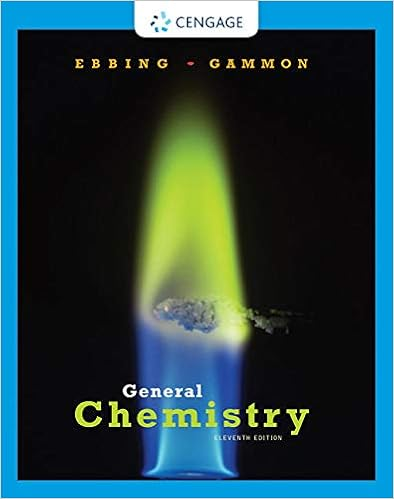Chem 2070 - CHEM 1007 Optional practice problems for the...

• Notes
• 7

This preview shows page 1 - 2 out of 7 pages.

We have textbook solutions for you!
The document you are viewing contains questions related to this textbook.The document you are viewing contains questions related to this textbook.
Chapter 9 / Exercise 9.81
General Chemistry
Ebbing/GammonExpert Verified
CHEM 1007: Optional practice problems for the second chem 2070 prelim exam 1. The first electron affinity of bromine (Br) releases 324.6 kJ/mole ( H = -324.6 kJ/mole ). The production of gaseous bromine ions from bromine molecules can be considered a two-step process in which the first step involves dissociating Br 2 (g) to make 2Br(g), requiring 193 kJ/mole. Br 2 (g) 2Br(g) H = +193 kJ/mole Does the formation of Br - (g) from Br 2 (g) release energy (exothermic) or require added energy (endothermic)? How much energy is added or released in the process of producing one mole of Br (g)? 2. Given the table of electron affinities and ionization energies below Element First ionization energy First electron affinity Ion Radius Chlorine (Cl) -0.580 aJ 133 pm Sodium (Na) 0.83 aJ 102 pm (a) Calculate the energy released in the following process: Na(g) + Cl(g) Na + (g) + Cl - (g) (b) Calculate the energy released in the following process: Na(g) + Cl(g) Na + Cl - (g) 3. Using the table of values in problem #2. When sodium chloride (NaCl) is heated in a flame, the flame takes on a yellow color. Calculate the energy associated with this reaction, shown below. The reaction that occurs in the gaseous state is: Na + (g) + Cl - (g) Na(g) + Cl(g) Find the wavelength of light that emits this energy in one photon. 4. Draw an MO energy diagram and predict the bond order for each of the following: Be 2 , Be 2 + and Be 2 - . Do you expect each of these molecules to exist in the gas phase? 5. Sketch the bonding molecular orbital that results from the linear combination of two 1s orbitals. Indicate the region where interference occurs and state the kind of interference (constructive or destructive). 6. Sketch the bonding and antibonding orbitals that result from the overlaps of the p z atomic orbitals in a homonuclear diatomic molecule. (Assume that the p z orbitals are those whose lobes are oriented along the bonding axis.) 7. -
We have textbook solutions for you!
The document you are viewing contains questions related to this textbook.The document you are viewing contains questions related to this textbook.
Chapter 9 / Exercise 9.81
General Chemistry
Ebbing/GammonExpert Verified
•••